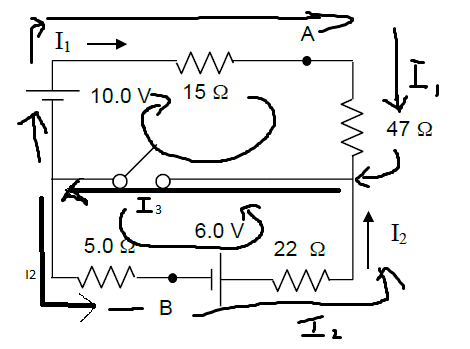# Circuit problem Kirchkoff's law

• johnknee
In summary, current passes through the closed switch and the power dissipated in the 47 ohm resistor is 0.383A.f

## Homework Statement

a) With the switch open, what is the current I1 ? (A positive sign means that current
flows in the direction of the arrow.)

b) Once the switch is closed in the circuit, what is the power dissipated in the 47 Ω
resistor?

c) How much current passes through the closed switch?

d) With the switch closed, what is the voltage difference, VA-VB ?

## Homework Equations

junction rule: I3 = I2 + I1
Loop rule(s)

## The Attempt at a Solution

I have attempted all 4 parts, please check my solutions? (parts a to c should be correct, but d not too sure.)

For a) Uses the same picture as part b

I believe that because there is a open switch, then the whole circuit became a series.
1st step: I got the Req value = 5ohms + 22ohms + 47ohms + 15ohms = 89ohms.
2nd step: I got the Veq value = 10.0V + (-6.0V) = 4.0v
3rd step: I did I = V/R = 4.0V/89ohms = 0.0449 A = I1.

For b) I have very badly drawn out all of the current directions(the switch is also closed) the top loop and the bottom loop would be separate with a junction at the middle piece on either end.First, I got that I2 + I1 = I3.

Then, I solved for the top loop, starting at the negative terminal side of the battery with 10V moving clockwise.
Top loop(clockwise): +10V1 - 15*I1 - 47*I1 + (0V since I'm assuming no Voltage drop across switch) = 0
Bottom loop(counter clockwise): -5*I2 + 6V2 - 22*I2 + 0V = 0

For the top loop: I isolated I1 and got 10/62 = 0.161 = I1
For the bottom loop: I got 6/27 = 0.222A = I2

Power dissipated in 47 ohm resistor I am assuming would be I1^2 * R = (0.161)^2 * 47 = 1.22 Watts?

Part c)
I'm not totally sure how I do this one, but I think that the current passing through the closed switch is just I3? which is I3 = I1 + I2 = 0.161 + 0.222 = 0.383A.

Part d)
For this part.. I am not quite sure.
I was thinking of like, VA - VB means we have to get from VB to VA in the circuit.
So... like VA - VB = +6V2 - 22*I2 + 47*I1? (going counter clockwise up the loop from the 6V battery.)
which would give me VA - VB = 6 - 22(0.222)+47(0.161) = 8.68 V.

Last edited:
That all looks right.